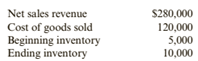Chapter 6, Problem 38BE### Cornerstones of Financial Accounti...

4th Edition
Jay Rich + 1 other
ISBN: 9781337690881

#### Solutions

Chapter
Section### Cornerstones of Financial Accounti...

4th Edition
Jay Rich + 1 other
ISBN: 9781337690881
Textbook Problem
1 views

# Inventory AnalysisCallahan Company reported the following information for the current year.Required:1. Compute Callahan’s (a) gross profit ratio, (b) inventory turnover ratio, and (c) average days to sell inventory. (Round all answers to two decimal places.)2. Explain the meaning of each number.

To determine

Concept introduction:

Gross Profit ratio:

Gross profit ratio is calculated by dividing the gross profit by sales. The formula to calculate the gross profit ration is as follows:

Gross Profit = Gross ProfitSales

Inventory Turnover Ratio:

Inventory Turnover Ratio measures the efficiency of the company in converting its inventory into sales. It is calculated by dividing the Cost of goods sold by Average inventory. The formula of the Inventory Turnover Ratio is as follows:

Inventory Turnover Ratio=Cost of goods soldAverage inventory

Note: Average inventory is calculated with the help of following formula:

Average inventory=(Beginning inventory + Ending inventory)2

Requirement 1:

To calculate:

The Gross Profit Ratio, Inventory Turnover ratio, and Average days to sell inventory.

Explanation

The Gross Profit Ratio, Inventory Turnover ratio, and Average days to sell inventory are calculated as follows:

 Net Sales (A) $280,000 Cost of Goods sold (B)$ 120,000 Gross Profit (C) = (A-B) \$ 160,000 Gross Profit Ratio (D) = (C/A) 57...
To determine

Concept introduction:

Gross Profit ratio:

Gross profit ratio is calculated by dividing the gross profit by sales. The formula to calculate the gross profit ration is as follows:

Gross Profit = Gross ProfitSales

Inventory Turnover Ratio:

Inventory Turnover Ratio measures the efficiency of the company in converting its inventory into sales. It is calculated by dividing the Cost of goods sold by Average inventory. The formula of the Inventory Turnover Ratio is as follows:

Inventory Turnover Ratio=Cost of goods soldAverage inventory

Note: Average inventory is calculated with the help of following formula:

Average inventory=(Beginning inventory + Ending inventory)2

Requirement 2:

To explain:

The Gross Profit Ratio, Inventory Turnover ratio, and Average days to sell inventory.

### Still sussing out bartleby?

Check out a sample textbook solution.

See a sample solution

#### The Solution to Your Study Problems

Bartleby provides explanations to thousands of textbook problems written by our experts, many with advanced degrees!

Get Started

#### What is logical independence?

Database Systems: Design, Implementation, & Management

#### What is a hybrid firewall?

Principles of Information Security (MindTap Course List)

#### What is a task? What is an event? What is a milestone?

Systems Analysis and Design (Shelly Cashman Series) (MindTap Course List)

#### Determine the rope tension T for which the pulley will be in equilibrium.

International Edition---engineering Mechanics: Statics, 4th Edition

#### What are three popular wearable devices?

Enhanced Discovering Computers 2017 (Shelly Cashman Series) (MindTap Course List)